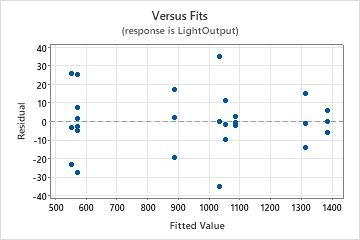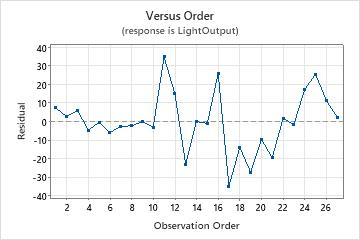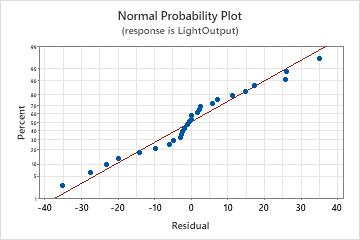# Interpret the key results for Balanced ANOVA

Complete the following steps to interpret a balanced ANOVA. Key output includes the p-value, group means, R2, and residual plots.

## Step 1: Determine whether the association between the response and the term is statistically significant

To determine whether the association between the response and each term in the model is statistically significant, compare the p-value for the term to your significance level to assess the null hypothesis. The null hypothesis is that there is no association between the term and the response. Usually, a significance level (denoted as α or alpha) of 0.05 works well. A significance level of 0.05 indicates a 5% risk of concluding that an association exists when there is no actual association.
P-value ≤ α: The association is statistically significant
If the p-value is less than or equal to the significance level, you can conclude that there is a statistically significant association between the response variable and the term.
P-value > α: The association is not statistically significant
If the p-value is greater than the significance level, you cannot conclude that there is a statistically significant association between the response variable and the term. You may want to refit the model without the term.
If there are multiple predictors without a statistically significant association with the response, you can reduce the model by removing terms one at a time. For more information on removing terms from the model, go to Model reduction.
For this analysis in Minitab, the model must be hierarchical. In a hierarchical model, all lower-order terms that comprise the higher-order terms also appear in the model. For example, a model that includes the interaction term A*B*C is hierarchical if it includes these terms: A, B, C, A*B, A*C, and B*C.
If a model term is statistically significant, the interpretation depends on the type of term. The interpretations are as follows:
• If a fixed factor is significant, you can conclude that not all the level means are equal.
• If a random factor is significant, you can conclude that the factor contributes to the amount of variation in the response.
• If an interaction term is significant, the relationship between a factor and the response depends on the other factors in the term. In this case, you should not interpret the main effects without considering the interaction effect.

Use the Means table to understand the statistically significant differences between the factor levels in your data. The mean of each group provides an estimate of each population mean. Look for differences between group means for terms that are statistically significant.

For main effects, the table displays the groups within each factor and their means. For interaction effects, the table displays all possible combinations of the groups. If an interaction term is statistically significant, do not interpret the main effects without considering the interaction effects.

## Step 2: Determine how well the model fits your data

To determine how well the model fits your data, examine the goodness-of-fit statistics in the Model Summary table.

S

Use S to assess how well the model describes the response. Use S instead of the R2 statistics to compare the fit of models that have no constant.

S is measured in the units of the response variable and represents how far the data values fall from the fitted values. The lower the value of S, the better the model describes the response. However, a low S value by itself does not indicate that the model meets the model assumptions. You should check the residual plots to verify the assumptions.

R-sq

The higher the R2 value, the better the model fits your data. R2 is always between 0% and 100%.

R2 always increases when you add additional predictors to a model. For example, the best five-predictor model will always have an R2 that is at least as high as the best four-predictor model. Therefore, R2 is most useful when you compare models of the same size.

Use adjusted R2 when you want to compare models that have different numbers of predictors. R2 always increases when you add a predictor to the model, even when there is no real improvement to the model. The adjusted R2 value incorporates the number of predictors in the model to help you choose the correct model.

Consider the following points when you interpret the R2 values:
• Small samples do not provide a precise estimate of the strength of the relationship between the response and predictors. For example, if you need R2 to be more precise, you should use a larger sample (typically, 40 or more).

• Goodness-of-fit statistics are just one measure of how well the model fits the data. Even when a model has a desirable value, you should check the residual plots to verify that the model meets the model assumptions.

## Step 3: Determine whether your model meets the assumptions of the analysis

Use the residual plots to help you determine whether the model is adequate and meets the assumptions of the analysis. If the assumptions are not met, the model may not fit the data well and you should use caution when you interpret the results.

For more information on how to handle patterns in the residual plots, go to Residual plots for Fit General Linear Model and click the name of the residual plot in the list at the top of the page.

### Residuals versus fits plot

Use the residuals versus fits plot to verify the assumption that the residuals are randomly distributed and have constant variance. Ideally, the points should fall randomly on both sides of 0, with no recognizable patterns in the points.

The patterns in the following table may indicate that the model does not meet the model assumptions.
Pattern What the pattern may indicate
Fanning or uneven spreading of residuals across fitted values Nonconstant variance
Curvilinear A missing higher-order term
A point that is far away from zero An outlier
A point that is far away from the other points in the x-direction An influential point
In this residuals versus fits plot, the data appear to be randomly distributed about zero. There is no evidence that the value of the residual depends on the fitted value.### Residuals versus order plot

Use the residuals versus order plot to verify the assumption that the residuals are independent from one another. Independent residuals show no trends or patterns when displayed in time order. Patterns in the points may indicate that residuals near each other may be correlated, and thus, not independent. Ideally, the residuals on the plot should fall randomly around the center line:If you see a pattern, investigate the cause. The following types of patterns may indicate that the residuals are dependent.
In this residuals versus order plot, the residuals appear to fall randomly around the centerline. There is no evidence that the residuals are not independent.### Normal probability plot

Use the normal probability plot of the residuals to verify the assumption that the residuals are normally distributed. The normal probability plot of the residuals should approximately follow a straight line.

The patterns in the following table may indicate that the model does not meet the model assumptions.
Pattern What the pattern may indicate
Not a straight line Nonnormality
A point that is far away from the line An outlier
Changing slope An unidentified variable
In this normal probability plot, the points generally follow a straight line. There is no evidence of nonnormality, outliers, or unidentified variables.By using this site you agree to the use of cookies for analytics and personalized content.  Read our policy